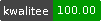# NAME

Bag::Similarity - Similarity measures for bags# SYNOPSIS

``  use Bag::Similarity;``

# DESCRIPTION

Bag::Similarity is the base class for similarity measures of bags.

# METHODS

All methods can be used as class or object methods.

## new

``  \$object = Bag::Similarity->new();``

## similarity

``  my \$similarity = \$object->similarity(\$any1,\$any1,\$width);``

`\$any` can be an arrayref, a hashref or a string. Strings are tokenized into n-grams of width `\$width`.

`\$width` must be integer, or defaults to 1.

## from_tokens

``  my \$similarity = \$object->from_tokens(['a','b'],['b']);``

## from_bags

``  my \$similarity = \$object->from_bags(['a'],['b']);``

Croaks if called directly. This method should be implemented in a child module.

## intersection

``  my \$intersection_size = \$object->intersection(['a'],['b']);``

## combined_length

``  my \$set_size_sum = \$object->combined_length(['a'],['b']);``

## min

``  my \$min_set_size = \$object->min(['a'],['b']);``

## ngrams

``````  my @monograms = \$object->ngrams('abc');
my @bigrams = \$object->ngrams('abc',2);``````

## _any

``  my \$arrayref = \$object->_any(\$any,\$width);``

# AUTHOR

Helmut Wollmersdorfer <helmut@wollmersdorfer.at>